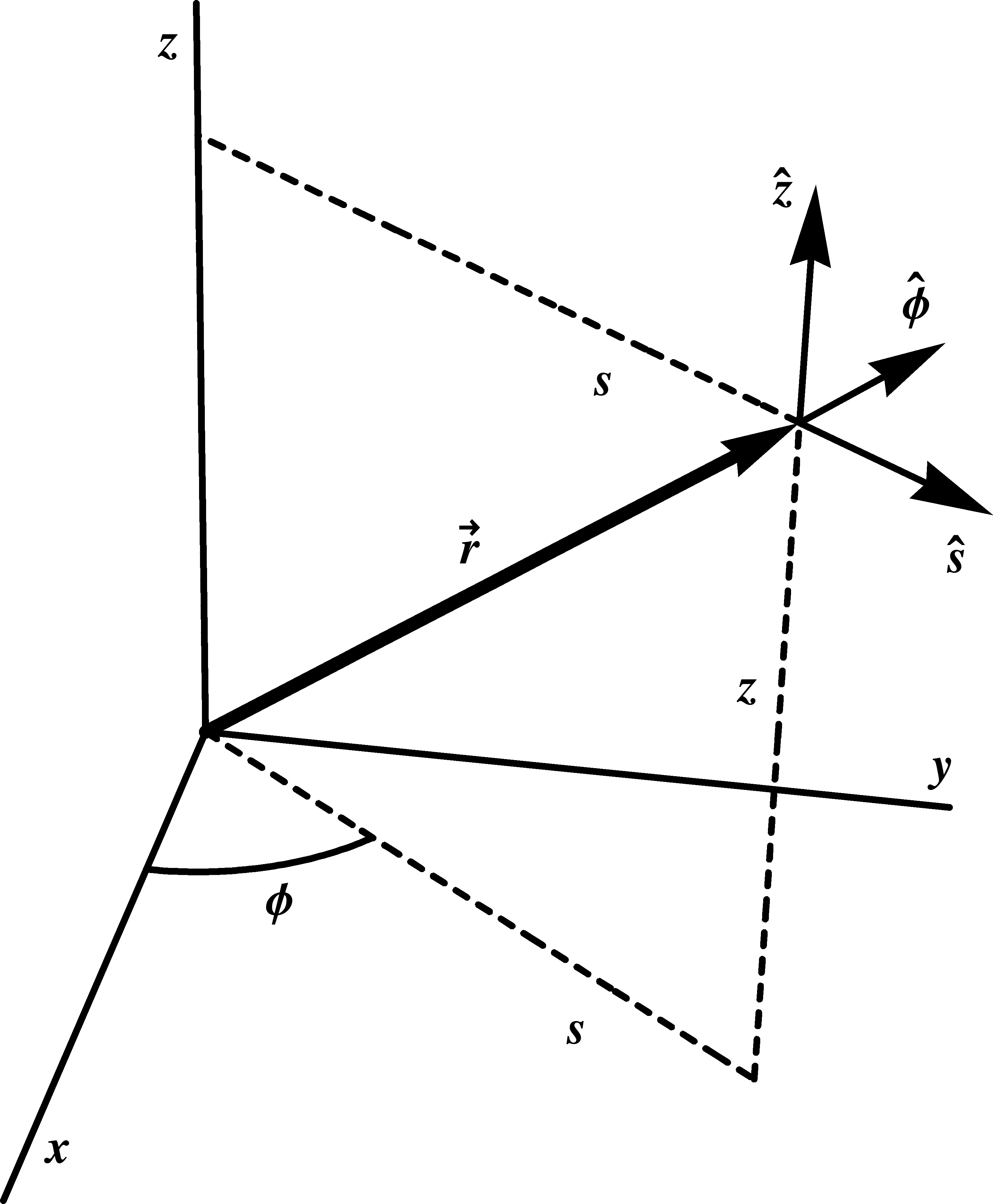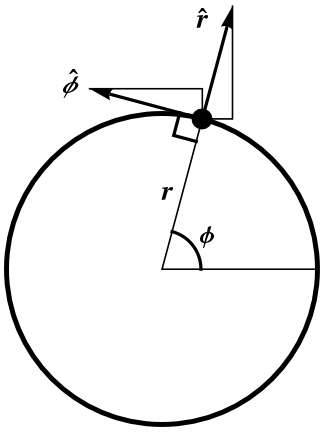## Section1.11Orthonormal Basis Vectors

In (1.6.1), we expressed an arbitrary vector $$\ww$$ in three dimensions in terms of the rectangular basis $$\{\xhat,\yhat,\zhat\}\text{.}$$ We have adopted the physics convention of writing unit vectors (i.e. vectors with magnitude one) with hats, rather than with arrows. You may find this to be a useful mnemonic.

When using curvilinear coordinates (see Section 1.2) it is often useful to expand the vector in terms of unit vectors associated with the curvilinear coordinates instead of the rectangular basis vectors above. For example, just as $$\xhat$$ points in the direction in which $$x$$ increases, $$\rhat$$ is defined to be the unit vector pointing in the direction of increasing $$r$$ (with the other coordinates held fixed).  1

In general, a basis vector of the form $$\widehat{coordinate}$$ is the unit vector that points in the direction in which $$coordinate$$ is changing. However, unlike the rectangular basis vectors, these vector fields vary from point to point.

### Activity1.11.1.Curvilinear Basis Vectors.

Imagine that the origin of coordinates is behind you in the corner of the room you are sitting in, on the floor, on the left-hand side. Think of your right shoulder as a point in space. You should use your right arm to represent, successively, each of the basis vectors $$\xhat\text{,}$$ $$\yhat\text{,}$$ and $$\zhat$$ in rectangular coordinates, $$\shat\text{,}$$ $$\phat\text{,}$$ and $$\zhat$$ in cylindrical coordinates and $$\rhat\text{,}$$ $$\that\text{,}$$ and $$\phat$$ in spherical coordinates.

Hint.Figure 1.11.1. The definition of cylindrical and spherical coordinates, showing the associated basis vectors.

In this activity, you should have learned the following things.

1. Figure 1.11.1 shows the directions that you should have pointed if you were standing at a particular position in the room. Make sure that the directions in which you pointed agree with the directions shown in the figure. In particular, $$\that$$ should point generally downward (for $$z>0$$) since $$\theta$$ is measured from the positive $$z$$-axis.

2. The basis vectors are vectors, that is, they are straight arrows in space, even when they correspond to coordinates that are angles. Your arm should therefore have been straight, no matter which basis vector you were representing.

3. Although the basis vectors that correspond to rectangular coordinates $$x\text{,}$$ $$y\text{,}$$ and $$z$$ are constants, and point in the same direction at each point in space, most of the basis vectors that correspond to cylindrical and spherical coordinates point in different directions at different points in space. Figure 1.11.2 shows the construction of the polar (or cylindrical) basis vectors $$\rhat$$ and $$\phat$$ at a particular point in a way that details how the direction of these vectors depends on the coordinate $$\phi$$ of the point.

4. The basis vectors adapted to a single coordinate form a simple example of the geometrical notion of a vector field, that is, a vector at every point in space, see Section 1.18. As an example, Figure 1.18.1 shows these basis vector fields in polar coordinates.Figure 1.11.2. The construction of the polar basis $$\{\rhat,\phat\}$$ at a specific point in the plane.
In some of our past materials, we have used a convention in which $$r$$ and $$\rhat$$ refer to geometrically different quantities in cylindrical than in spherical coordinates. If it is not obvious from the context (it usually is), care must be taken to specify in which coordinate system one is working.